# JUDEA PEARL

## The Art and Science of Causal Effect (Part 2)

### SLIDE 40: OUTLINE - PART 2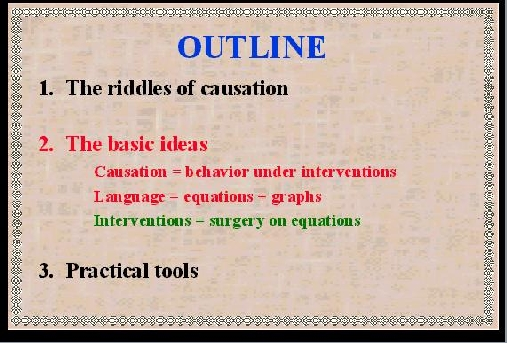This brings us to the second part of the lecture: how the second riddle of causation can be solved by combining equations with graphs, and how this solution makes the first riddle less formidable. The overriding ideas in this solution are: FIRST: treating causation as a summary of behavior under interventions and SECOND: using equations and graphs as a mathematical language within which causal thoughts can be represented and manipulated. And to put the two together, we need a THIRD concept: Treating interventions a s a surgery over equations. Let us start with an area that uses causation extensively and never had any trouble with it: Engineering.

### SLIDE 41: CIRCUIT DIAGRAM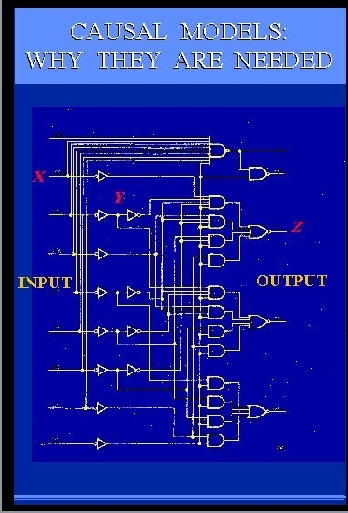Here is an engineering drawing of a circuit diagram that shows cause-effect relations among the signals in the circuit. The circuit consists of AND gates and OR gates, each performing some logical function between input and output. Let us examine this diagram closely, since its simplicity and familiarity are very deceiving. This diagram is, in fact, one of the greatest marvels of science. It is capable of conveying more information than millions of algebraic equations or probability functions or logical expressions". What makes this diagram so much more powerful is the ability to predict not merely how the circuit behaves under normal conditions, but also how the circuit will behave under millions of ABNORMAL conditions. For example, given this circuit diagram, we can easily tell what the output will be if some input changes from 0 to 1. This is normal and can easily be expressed by a simple input-output equation. Now comes the abnormal part. We can also tell what the output will be when we set Y to 0 (zero), or tie it to X, or change this AND gate to an OR gate, or when we perform any of the millions combinations of these operations.
The designer of this circuit did not anticipate or even consider such weird interventions, yet, miraculously, we can predict their consequences. How? Where does this representational power come from?

It comes from what early economists called AUTONOMY, namely, the gates in these diagram represent independent mechanisms - it is easy to change one without changing the other. The diagram takes advantage of this independence and describes the normal functioning of the circuit USING PRECISELY THOSE BUILDING BLOCKS THAT WILL REMAIN UNALTERED UNDER INTERVENTION.

My colleagues from Boelter Hall are surely wondering why I stand here before you blathering about an engineering triviality as if it were the 8th wonder of the world. I have three reasons for doing this. First, I will try to show that there is a lot of unexploited wisdom in practices that engineers take for granted.

### SLIDE 42: PATH DIAGRAMS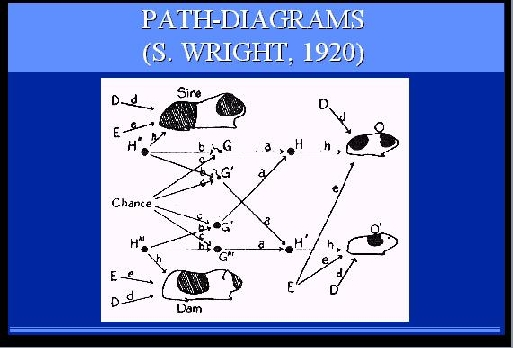Second, I am trying to remind economists and social scientists of the benefits of this diagrammatic method. They have been using a similar method on and off for over 75 years, called structural equations modeling and path-diagrams, but in recent years have allowed algebraic convenience to suppress the diagrammatic representation, together with its benefits. Finally, these diagrams capture in my opinion, the very essence of causation - the ability to predict the consequences of abnormal eventualities and new manipulations. In this diagram, for example, it is possible to predict what coat pattern the litter guinea-pig is likely to have, if we change environmental factors, shown here by as input (E) in green, or even genetic factors, shown in red as intermediate nodes between parents
and offsprings (H). Such predictions cannot be made on the basis of algebraic or correlational analysis.

Viewing causality this way explains why scientists pursue causal explanations with such zeal, and why attaining a causal model is accompanied with a sense of gaining "deep understanding" and "being in control."

### SLIDE 43: DUCK MACHINE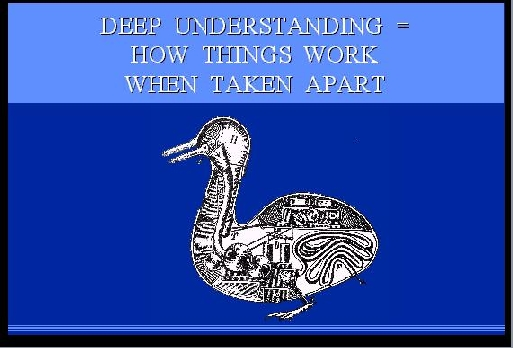DEEP UNDERSTANDING means knowing, not merely how things behaved yesterday, but also how things will behave under new hypothetical circumstances, control being one such circumstance. Interestingly, when we have such understanding we feel "in control" even when if we have no practical way of controlling things. For example, we have no practical way to control celestial motion, and still the theory of gravitation gives us a feeling of understanding and control, because it provides a blueprint for hypothetical control. We can predict the effect on tidal waves of unexpected new events, say, the moon being hit by a meteor or the gravitational constant suddenly diminishing by a factor of 2 and, just as important, the gravitational theory gives us the assurance that ordinary manipulation
of earthly things will NOT control tidal waves. It is not surprising that causal models are viewed as the litmus test distinguishing deliberate reasoning from reactive or instinctive response. Birds and monkeys may possibly be trained to perform complex tasks such as fixing a broken wire, but that requires trial-and-error training. Deliberate reasoners, on the other hand, can anticipate the consequences of new manipulations WITHOUT EVER TRYING those manipulations.

### SLIDE 44: EQUATIONS VS. DIAGRAMS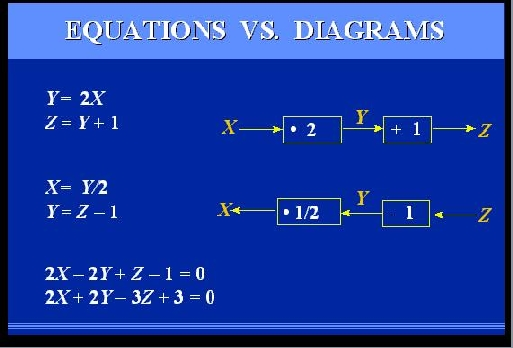Let us magnify a portion of the circuit diagram so that we can understand why the diagram can predict outcomes that equations can not. Let us also switch from logical gates to linear equations (to make everyone here more comfortable), and assume we are dealing with a system containing just two components: a multiplier and an adder. The MULTIPLIER takes the input and multiplies it by a factor of 2; the ADDER takes its input and adds a 1 to it. The equations describing these two components are given here on the left. But are these equations EQUIVALENT to the diagram on the right? Obviously not! If they were, then let us switch the variables around, and the resulting two equations should be equivalent to the circuit shown below. But these two circuits are different.
The top one tells us that if we physically manipulate Y it will affect Z, while the bottom one shows that manipulating Y will affect X and will have no effect on Z. Moreover, performing some additional algebraic operations on our equations, we can obtain two new equations, shown at the bottom, which point to no structure AT ALL; they simply represent two constraints on three variables, without telling us how they influence each other.

Let us examine more closely the mental process by which we determine the effect of physically manipulating Y, say setting Y to 0.

### SLIDE 45: INTERVENTION AS SURGERY ON MECHANISM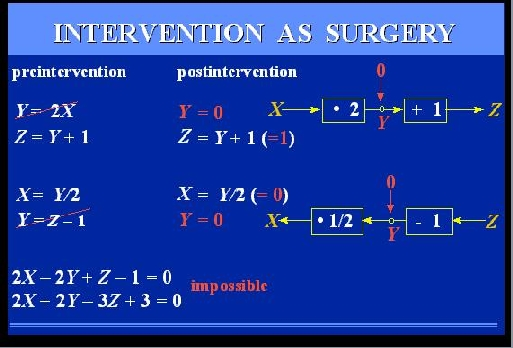Clearly, when we set Y to 0, the relation between X and Y is no longer given by the multiplier - a new mechanism now controls Y, in which X has no say. In the equational representation, this amounts to replacing the equation Y=2X by a new equation, Y=0, and solving a new set of equations, which gives Z = 1. If we perform this surgery on the lower pair of equations, representing to the lower model, we get of course a different solution. The second equation will need to be replaced, which will yield X = 0 and leave Z unconstrained. We now see how this model of intervention leads to a formal definition of causation: "Y is a cause of Z if we can change Z by manipulating Y, namely, if after surgically removing the equation for Y, the solution for Z will depend on the new value we
substitute for Y". We also see how vital the diagram is in this process. THE DIAGRAM TELLS US WHICH EQUATION IS TO BE DELETED WHEN WE MANIPULATE Y. That information is totally washed out when we transform the equations into algebraically equivalent form, as shown at the bottom of the screen - from this pair equations alone, it is impossible to predict the result of setting Y to 0, because we do not know what surgery to perform - there is no such thing as "the equation for Y".

IN SUMMARY, INTERVENTION AMOUNTS TO A SURGERY ON EQUATIONS, GUIDED BY A DIAGRAM, AND CAUSATION MEANS PREDICTING THE CONSEQUENCES OF SUCH A SURGERY.

This is a universal theme that goes beyond physical systems. In fact, the idea of modeling interventions by "wiping out" equations was first proposed by an ECONOMIST, Herman Wold in 1960, but his teachings have all but disappeared from the economics literature. History books attribute this mysterious disappearance to Wold's personality, but I tend to believe that the reason goes deeper: Early econometricians were very careful mathematicians; they fought hard to keep their algebra clean and formal, and could not agree to have it contaminated by gimmicks such as diagrams. And as we see on the screen the surgery operation makes no mathematical sense without the diagram, as it is sensitive to the way we write the equations.

Before expounding on the properties of this new mathematical operation, let me demonstrate how useful it is for clarifying concepts in statistics and economics.

### SLIDE 46: INTERVENTION AS SURGERY - CONTROLLED EXPERIMENTS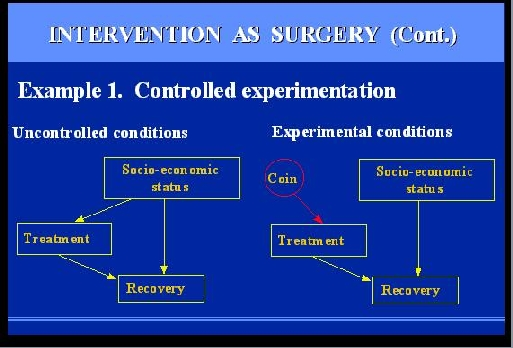Why do we prefer controlled experiment over uncontrolled studies? Assume we wish to study the effect of some drug treatment on recovery of patients suffering from a given disorder. The mechanism governing the behavior of each patient is similar in structure to the circuit diagram we saw earlier: Recovery is a function of both the treatment and other factors, such as socio-economic conditions, life-style, diet, age etc. only one such factor is shown here. Under uncontrolled conditions, the choice of treatment is up to the patients, and may depend on the patients socio-economic background. This creates a problem, because we can't tell if changes in recovery rates are due to treatment or to those background factors. What we wish to do is compare patients of same backgrounds and that is precisely what Fisher's RANDOMIZED EXPERIMENT accomplishes. How?
It actually consists of two parts, randomization and INTERVENTION. Intervention means that we change the natural behavior of the individual: we separate subjects into two groups, called treatment and control, and we convince the subjects to obey the experimental policy. We assign treatment to some patients who, under normal circumstances, will not seek treatment, and we give placebo to patients who otherwise would receive treatment. That, in our new vocabulary, means SURGERY - we are severing one functional link and replacing it by another. Fisher's great insight was that connecting the new link to a random coin flip, GUARANTEES that the link we wish to break, is actually broken. The reason is, that a random coin is assumed unaffected by anything we can measure on a macroscopic level, including, of course, a patient socio-economic background.

This picture provides a meaningful and formal rationale for the universally accepted procedure of randomized trials. In contrast, our next example uses the surgery idea to point out inadequacies in widely accepted procedures.

### SLIDE 47: EXAMPLE 2 - POLICY ANALYSIS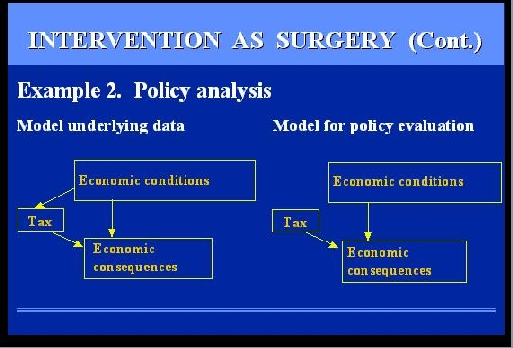The example involves a Government official trying to evaluate the economic consequences of some policy, say taxation. A deliberate decision to raise or lower taxes is a surgery on the model of the economy because it modifies the conditions prevailing while the model was built. Economic models are built on the basis of data taken over some period of time, and during this period of time, taxes were lowered and raised in response to some economic conditions or political pressure. However, when we EVALUATE a policy, we wish to compare alternative policies under the SAME economic conditions, namely we wish to sever this link that, in the past, has tied policies to those conditions.
In this set-up, it is impossible of course to connect our policy to a coin and run a controlled experiment; we do not have the time for that, and we might ruin the economy before the experiment is over. Nevertheless the analysis that we SHOULD CONDUCT is to infer the behavior of this mutilated model from data governed by a non-mutilated model.

I said, SHOULD CONDUCT, because you will not find such analysis in any economics textbook. As I mentioned earlier, the surgery idea of Herman Wold, was stamped out of the economics literature in the 1970's and all discussions on policy analysis that I could find, assume that the mutilated model prevails throughout. The fact that taxation is under government control at the time of evaluation is assumed to be sufficient for treating taxation an exogenous variable throughout when, in fact, taxation is an endogenous variable during the model-building phase, and turns exogenous only when evaluated. Of course, I am not claiming that reinstating the surgery model would enable the government to balance its budget overnight, but it is certainly something worth trying.

Let us examine now how the surgery interpretation resolves Russell's enigma: concerning the clash between the directionality of causal relations and the symmetry of physical equations. The equations of physics are indeed symmetrical, but when we compare the phrases "A CAUSES B" vs. "B CAUSES A" we are not talking about a single set of equations. Rather, we are comparing two world models, represented by two different sets of equations; one in which the equation for A is surgically removed, the other where the equation for B is removed. Russell would probably stop us at this point and ask: "How can you talk about TWO world models, when in fact there is only one world model, given by all the equations of physics put together?" The answer is: YES. If you wish to include the entire universe in the model, causality disappears because interventions disappear - the manipulator and the manipulated loose their distinction. However, scientists rarely consider the entirety of the universe as an object of investigation. In most cases the scientist carves a piece from the universe and proclaims that piece: IN namely, the FOCUS of investigation. The rest of the universe is then considered OUT or BACKGROUND, and is summarized by what we call BOUNDARY CONDITIONS. This choice of INs and OUTs creates asymmetry in the way we look at things, and it is this asymmetry that permits us to talk about "outside intervention", hence, causality and cause-effect directionality.

### SLIDE 48: HAND-EYE SYSTEM (DESCARTES, L'HOMME)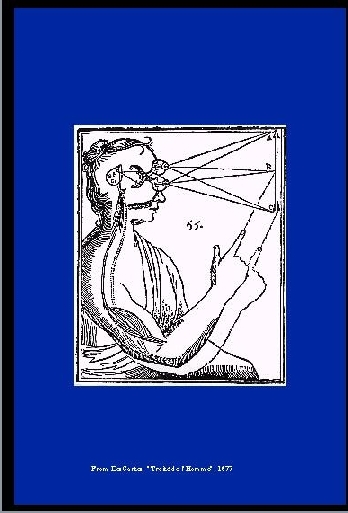This can be illustrated quite nicely using Descartes classical drawing. As a whole, this hand-eye system knows nothing about causation. It is merely a messy plasma of particles and photons trying their very best to obey Schroedinger's Equation, which is symmetric.

### SLIDE 49: HAND-EYE SECTION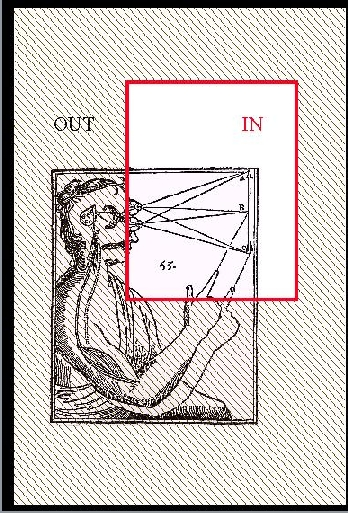However, carve a chunk from it, say the object part, and we can talk about the motion of the hand CAUSING this light ray to change angle.

### SLIDE 50: EYE-HAND SECTION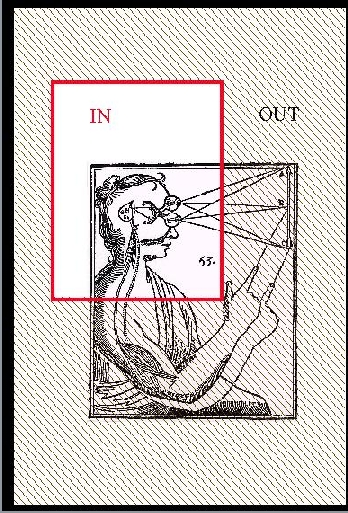Carve it another way, focusing on the brain part, and, lo and behold, it is now the light ray that causes the hand to move - precisely the opposite direction. The lesson is that it is the way we carve up the universe that determines the directionality we associate with cause and effect. Such carving is tacitly assumed in every scientific investigation. In artificial intelligence it was called circumscription, by J. McCarthy. In economics, circumscription amount to deciding which variables are deemed endogenous and which ones exogenous, IN the model or EXTERNAL to the model.

### SLIDE 51: FROM PHYSICS TO CAUSALITY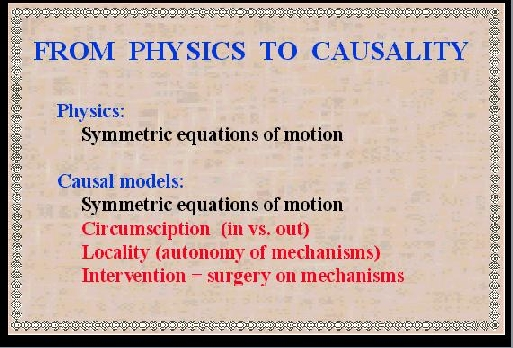Let us summarize the essential differences between equational and causal models. Both use a set of symmetric equations to describe normal conditions. The causal model, however, contains three additional ingredients: a distinction between the IN and the OUT. An assumption that each equation corresponds to an independent mechanism, hence, it must be preserved as a separate mathematical sentence. Interventions are interpreted as surgeries over those mechanism. This brings us closer to realizing the dream of making causality a friendly part of physics. But one ingredient is missing: THE ALGEBRA. We discussed earlier how important the computational facility of algebra was to scientists and engineers in the Galilean era. Can we expect such algebraic facility to serve causality as well? Let me rephrase it differently: Scientific activity, as we know it, consists of two basic components:

### SLIDE 53: HAMMERING A MAGNET (GILBERT, DE MAGNET, 1600)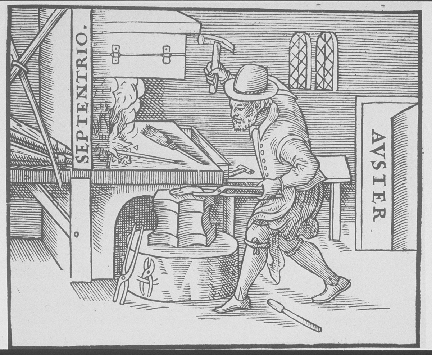and interventions.

### SLIDE 54: LABORATORY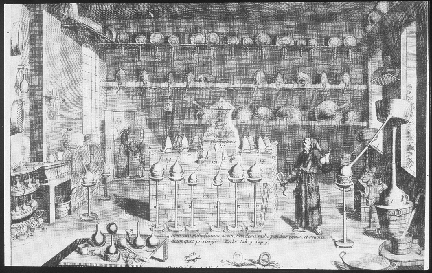The combination of the two is what we call a LABORATORY, a place where we control some of the conditions and observe others. It so happened that standard algebras have served the observational component very well, but, thus far, have not benefited the interventional component. This is true for the algebra of equations, Boolean algebra, and probability calculus, all are geared to serve observational sentences, but not interventional sentences.

### SLIDE 55: NEEDED: ALGEBRA OF DOING (CONT.)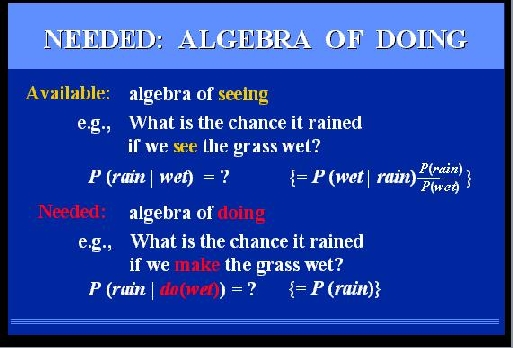Take for example, probability theory. If we wish to find the chance it rained, given that we see the grass wet, we can express our question in a formal sentence written like that: P(rain|wet) to be read: Probability~Of~Rain~given~~Wet. The vertical bar stands for the phrase: "given that we see". Not only can we express this question in a formal sentence but we can also use the machinery of probability theory and transform the sentence into other expressions. In our example, the sentence on the left can be transformed to the one on the right, if we find it more convenient or informative. But suppose we ask a different question: "What is the chance it rained if we MAKE the grass wet?" We cannot even express our query in the syntax of probability, because the vertical bar is already taken to mean "given that I see". We can invent a new symbol
"DO", and each time we see a DO after the bar we read it "GIVEN THAT WE DO" - but this does not help us compute the answer to our question, because the rules of probability do not apply to this new reading. We know intuitively what the answer should be: P(rain), because making the grass wet does not change the chance of rain. But can this intuitive answer, and others like it, be derived mechanically? so as to comfort our thoughts when intuition fails?

The answer is YES, and it takes a new algebra: First, we assign a symbol to the new operator "given that I do". Second, we find the rules for manipulating sentences containing this new symbol. We do that by a process analogous to the way mathematicians found the rules of standard algebra.

### SLIDE 56: NEEDED: ALGEBRA OF DOING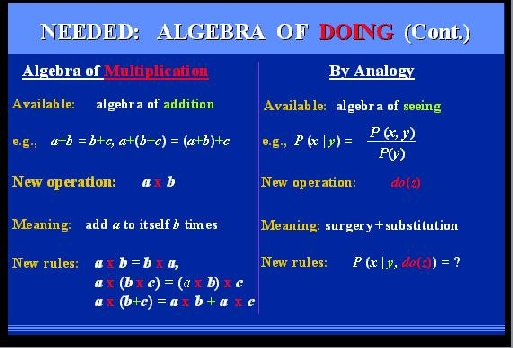Imagine that you are a mathematician in the 16th century, you are now an expert in the algebra of ADDITION, and you feel an urgent need to introduce a new operator: MULTIPLICATION, because you are tired of adding a number to itself all day long. First thing you do is assign the new operator a symbol: MULTIPLY. Then you go down to the meaning of the operator, from which you can deduce its rules of transformations. For example: the commutative law of multiplication can be deduced that way, the associative law, and so on,.... we now learn all this in high school. In exactly the same fashion, we can deduce the rules that govern our new symbol: do(x). We have an algebra for seeing, namely, probability theory. We have a new operator, with a brand new red outfit and a very clear meaning, given to us by the surgery procedure. The door is open for deduction and the result is give in the next slide.

### SLIDE 57: CAUSAL CALCULUS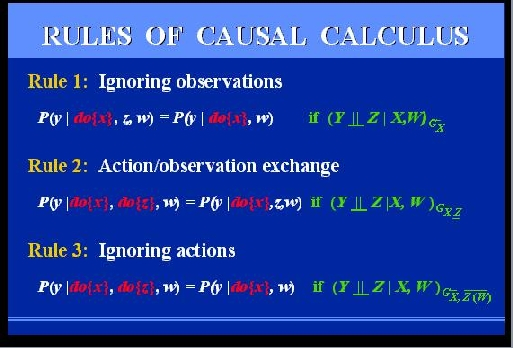Please do not get alarmed, I do not expect you to read these equations right now, but I think you can still get the flavor of this new calculus. It consist of 3 rules that permit us to transform expressions involving actions and observations, into other expressions of this type. The first allows us to ignore an irrelevant observation, the third to ignore an irrelevant action, the second allows us to exchange an action with an observation of the same fact. What are those green symbols on the right? These are the green lights which the diagram gives us, whenever the transformation is legal. We will see them in action on our next example.